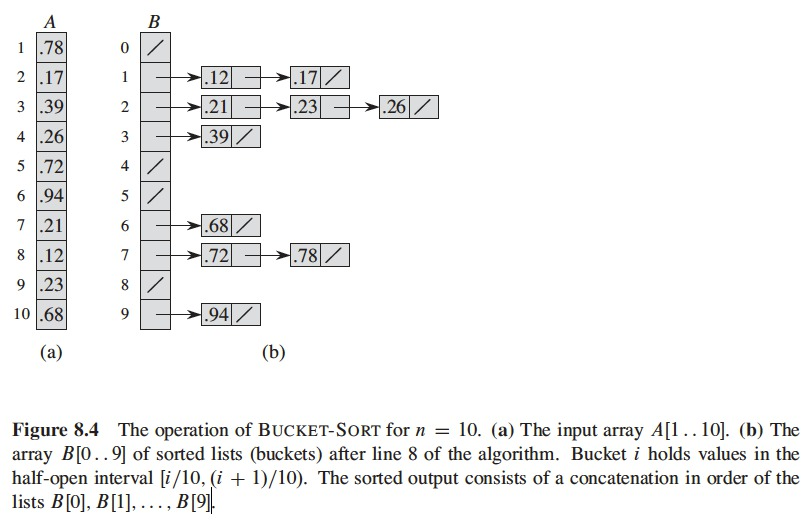July 17, 2019

# Sorting in Linear Time

• Previous sorting algorithms
• Were based on comparisons, comparison sort algorithms
• Can sort $$n$$ numbers in $$O(n \lg n)$$ time
• Merge sort and heapsort achieve this upper bound in the worst case
• Quicksort achieves this upper bound in the average case

# Lower Bounds for Sorting

• Comparison sort algorithms only use comparisons to get information about an input sequence $$\langle a_1, a_2, \dots, a_n \rangle$$
• One test: $$a_i < a_j$$, $$a_i \leq a_j$$, $$a_i = a_j$$, $$a_i \geq a_j$$, $$a_i > a_j$$, to determine order
• For the algorithms in this section
• Assume all input elements are distinct
• Comparisons $$a_i = a_j$$ are useless
• We only use comparisons of form $$a_i \leq a_j$$

# The Decision-tree Model

• Can view comparison sorts as decision trees
• Decision tree, a full binary tree representing the comparisons between elements performed by a particular sorting algorithm, given an input
• Control, data movement, other aspects of algorithm
• Ignored in the decision tree

# The Decision-tree Model

• Decision tree for the insertion sort algorithm
• Operates in a sequence of three elements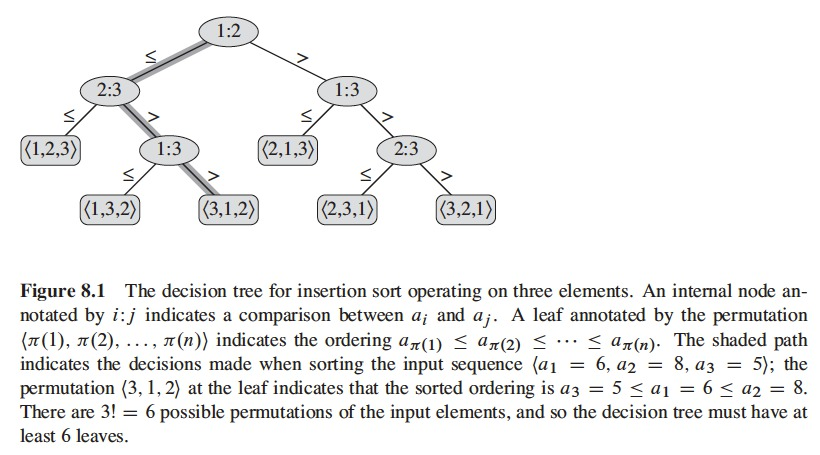# The Decision-tree Model

• Decision-tree Model
• Internal nodes annotated with $$i:j$$, $$1 \leq i$$, $$j \leq n$$, $$n$$ is the number of elements in the input sequence
• Leaf nodes annotated by a permutation $$\langle \pi(1), \pi(2), \dots, \pi(n) \rangle$$

# The Decision-tree Model

• Execution of the sorting algorithm
• Tracing a simple path from root down to leaf
• Each internal node indicates a comparison $$a_i \leq a_j$$
• Left subtree, $$a_i \leq a_j$$
• Right subtree, $$a_i > a_j$$
• When we reach a leaf, we have found the ordering of the elements $$a_{\pi(1)} \leq a_{\pi(2)} \leq \dots \leq a_{\pi(n)}$$
• Each of the $$n!$$ permutations must appear as a leaf for comparison sort to be correct
• Each leaf (permutation) must be reachable from the root node

# The Decision-tree Model

• Theorem 8.1
• Any comparison sort algorithm requires $$\Omega(n \lg n)$$ comparisons in the worst case.
• Why?
• Has to do with the height of the decision tree
• Permutaions (defining ordering) appear as reachable leafs
• Corolary 8.2
• Heapsort and merge sort are asymptotically optimal comparison sorts

# Counting Sort

• Assume input of $$n$$ integer elements in a range from 0 to $$k$$
• When $$k = O(n)$$, sorting runs in $$\Theta(n)$$
• Determine for each input element $$x$$, the number of elements smaller than $$x$$
• This is how it positions $$x$$ in its place in the output array
• If there are 17 elements smaller than $$x$$ $$\rightarrow$$ $$x$$ will be assigned position 18
• Must modify scheme in order to deal with repeated numbers

# Counting Sort

• $$A[j]$$, element of the original array
• $$C[A[j]]$$, number of repetitions of number in $$A[j]$$
• $$B[C[A[j]]]$$, final array, puts the number in its final position

# Counting Sort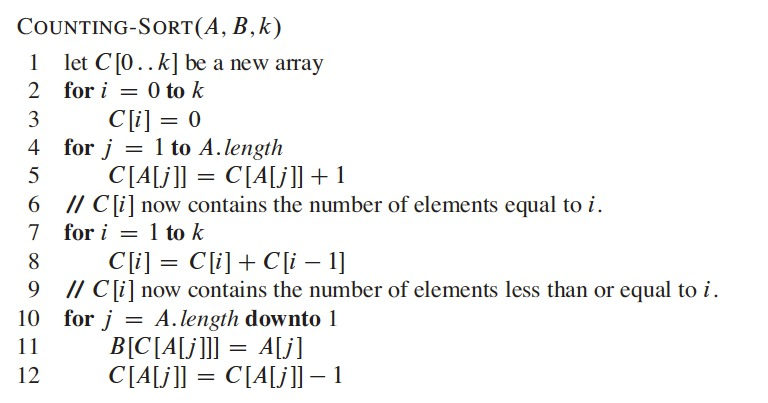# Counting Sort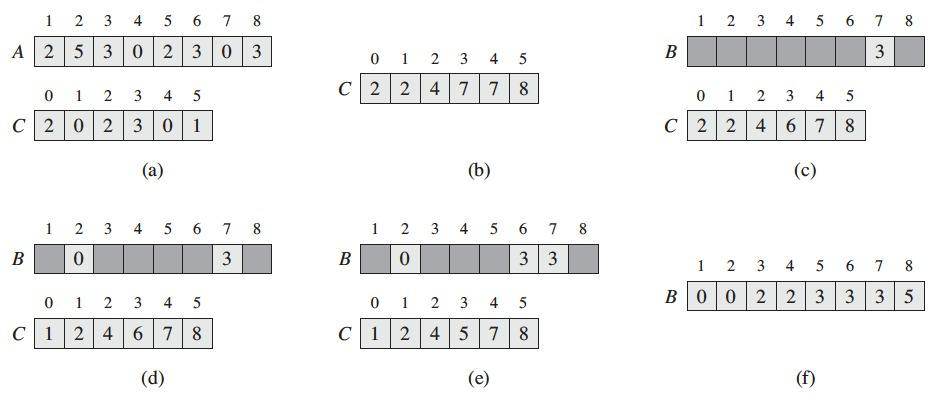# Counting Sort

• Lines 2-3 initialize array $$C$$ to zeros, $$\Theta(k)$$
• Lines 4-5, sets $$C[i]$$ to the number of elements equal to $$i$$, $$\Theta(n)$$
• Lines 7-8, determine for each $$i = 0, 1, \dots, k$$, how many input elements are less than or equal to $$i$$, keeping a running sum of the array $$C$$, $$\Theta(k)$$
• Lines 10-12, places each element $$A[j]$$ into its correct sorted position in $$B$$, $$\Theta(n)$$

# Counting Sort

• Counting sort beats the lower bound of $$\Omega(n \lg n)$$
• It is not a comparison sort
• It uses the values of the elements to index into an array
• $$\Omega(n \lg n)$$ doesn’t apply, it is not a comparison sort model

# Counting Sort

• Important property
• Counting sort is stable
• Numbers with the same value appear in the output array in the same order as they do in the input array
• Important when satellite data is moved around with sorted elements
• Used as subroutine of radix sort, important property for radix sort to be correct

• Radix Sort was originally used by card-sorting machines (IBM)
• Cards have 80 columns, each column has 12 places, a machine punches one of those holes
• Card-sorting machines worked with one column at a time
• Currently, radix sort is used for multi-key sorting (i.e. year/month/day)
• Considers each digit of the number as an independent key

• IBM punched cards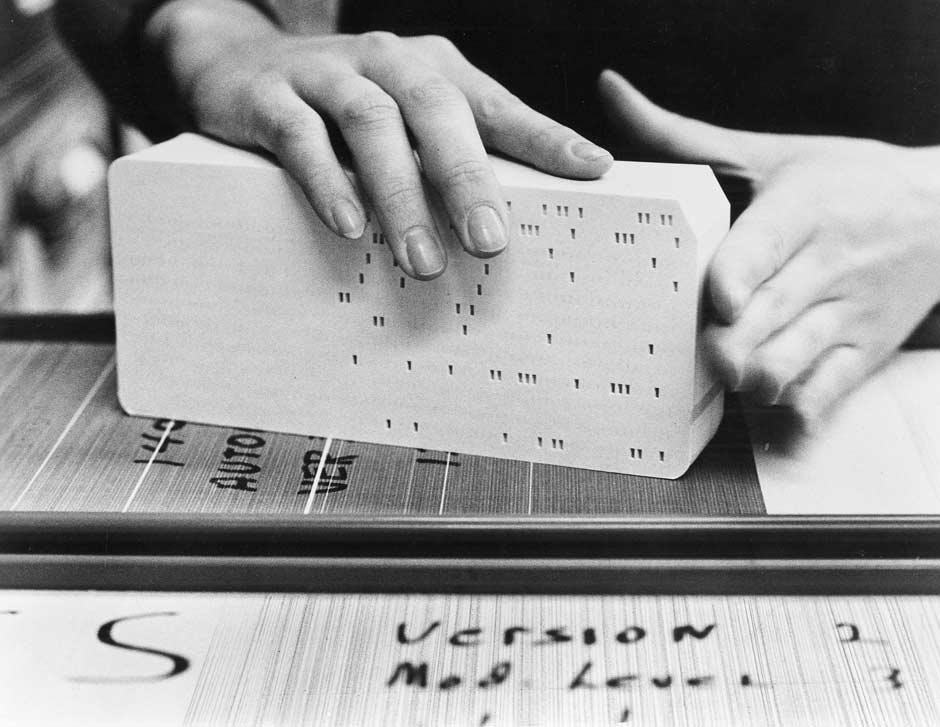http://www-03.ibm.com/ibm/history/ibm100/us/en/icons/punchcard/breakthroughs/

• IBM cards sorterhttps://en.wikipedia.org/wiki/IBM_card_sorter#/media/File:Punch_card_sorter.JPG

• How do we sort?
• Intuitively, we would sort numbers by the most significant digit, sort each of the resulting bins recursively, then combine the decks in order
• Problem
• the cards in 9 of the 10 bns must be put aside to sort each of the bins
• this procedure generates many intermediate piles of cards
• We would have to keep track of these piles

• How do we sort?
• Radix sort works from the least significant digit first
• It combines the cards into a single deck
• Cards in the 0 bin precede cards in the 1 bin which precede the cards in the 2 bin, and so on
• Then sorts the entire deck again on second least significant digit and recombines the deck
• Process continues until cards have been sorted on all $$d$$ digits
• Radix sort requires $$d$$ passes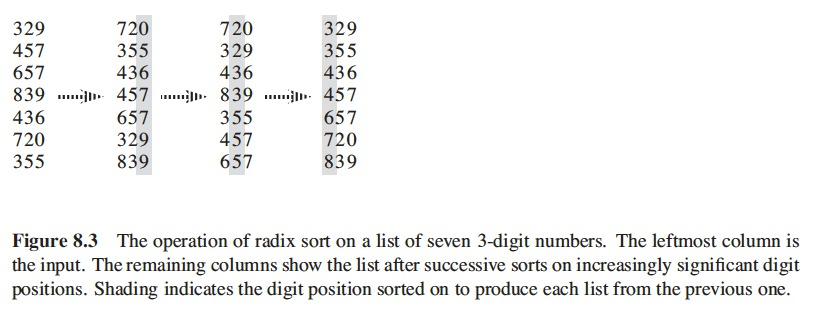• $$d$$ is the number of digits
• Digit $$1$$ has the lowest order
• Digit $$d$$ has the highest order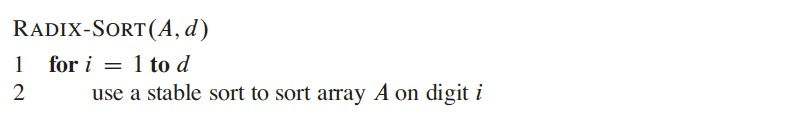• If each digit is in the range from 1 to $$k$$, we use CountingSort
• Each step for a digit takes $$\Theta(n+k)$$
• For $$d$$ digits $$\Theta(dn + dk)$$
• If $$d$$ is a constant and $$k = O(n)$$, $$T(n) = O(n)$$
• Radix-$$n$$ means that each digit can differentiate among $$n$$ different symbols, in previous examples we used radix-10 (digits from 0 to 9).

# Bucket Sort

• Bucket sort runs in linear time when the input elements are drawn from a uniform distribution, average-case running time $$O(n)$$
• Fast because it assumes something about the input
• (i.e. Counting sort assumes numbers in a small range)
• Bucket sort assumes numbers randomly generated and uniformly distributed in a range $$[0,1)$$

# Bucket Sort

• Divides the interval $$[0,1)$$ in $$n$$ sub-intervals (or buckets) of the same size
• Numbers distributed in buckets
• Don’t expect many numbers to fall in each bucket (they are uniformly and independently distributed over $$[0,1)$$)
• To sort the numbers, we sort numbers in each bucket, then go through buckets in order

# Bucket Sort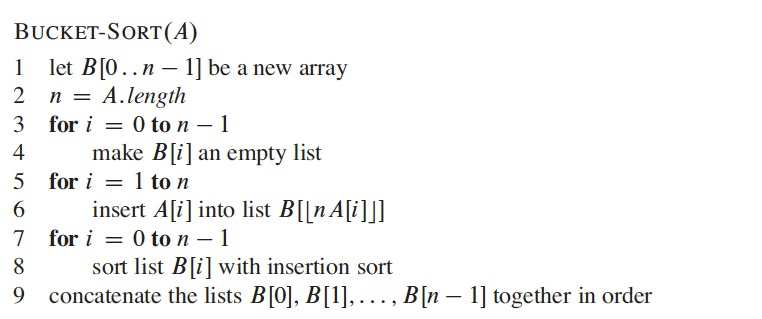# Bucket Sort